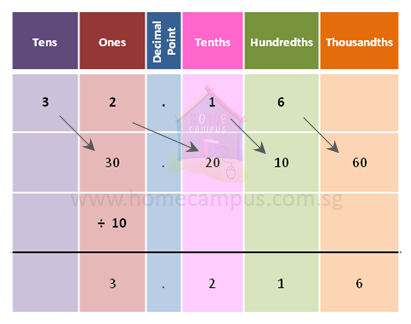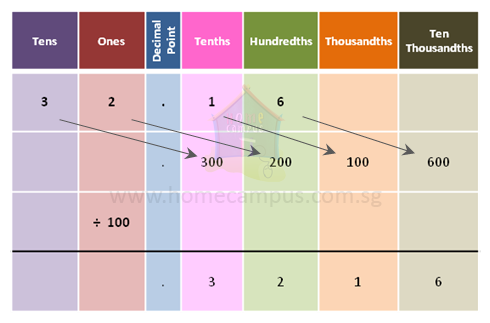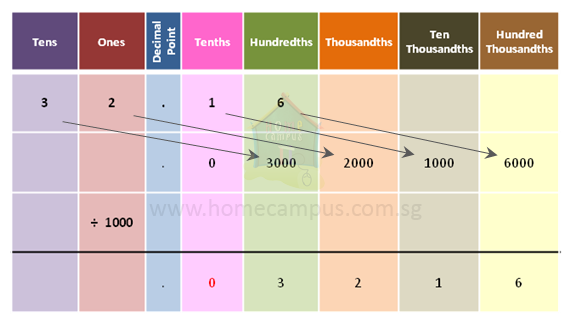## How to Divide Decimal Numbers by 10s, 100s and 1000s (Powers of 10)?

Practice Unlimited Questions

#### 1.  What is 32.16 divided by 10?32.16  ÷  10  =  3.216
When you divide a decimal number by 10, its decimal point moves 1 place to the left.

Quick trick: Since there is 1 zero in 10, move the decimal point 1 place to the left.

#### 2.  What is 32.16 divided by 100?32.16  ÷  100  =  0.3216
When you divide a decimal number by 100, its decimal point moves 2 places to the left.

Quick trick: Since there are 2 zeroes in 100, move the decimal point 2 places to the left.

#### 3.  What is 32.16 divided by 1000?32.16  ÷  1000  =  0.03216
When you divide a decimal number by 1000, its decimal point moves 3 places to the left.

Quick trick: Since there are 3 zeroes in 1000, move the decimal point 3 places to the left.

#### 4.  What is 32.16 divided by 200?

32.16  ÷  200
=  32.16  ÷  2  ÷  100
=  16.08  ÷  100
=  0.1608
32.16  ÷  2  =  16.08